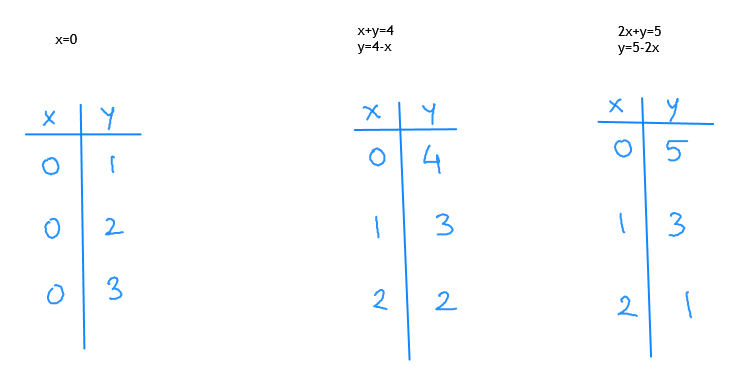# Graph the solution set of each system if inequalities.  X is greater than or = 0X+Y is less than or equal to 42x+y is less than or equal to 5

Question
18 views

Graph the solution set of each system if inequalities.

X is greater than or = 0

X+Y is less than or equal to 4

2x+y is less than or equal to 5

check_circle

Step 1

For the inequalities we make auxiliary equations.

x=0 , x+y=4, 2x+y=5

Step 2

Then we make table for the equations.help_outlineImage Transcriptioncloseх*у-4 y-4-x 2x-у-5 у-5-2х x-0 4 3 3 2 O 3 2 2 2 fullscreen
Step 3

Then plot the points and joi...

### Want to see the full answer?

See Solution

#### Want to see this answer and more?

Solutions are written by subject experts who are available 24/7. Questions are typically answered within 1 hour.*

See Solution
*Response times may vary by subject and question.
Tagged in

### Other